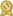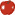Found 20 differential equations tutors in Chandler, AZ 85224 – page 1 of 2.

Phoenix 85001 · 15 miles from 85224 · \$20/hour · teaches Differential Equations

I have 6+ years tutoring experience. My main goal is to impart the knowledge I have experienced to those who are in need. The knowledge I gained through my experience have always helped me to assist students in many ways.Red Apple award, certified, member for 5 years and 9 months

Chandler 85286 · 3 miles from downtown · \$60/hour · teaches Differential

...I've had to take pretty much every class from Differential Equations to Philosophy, and everything in between. Trust me, I've been exactly where you are right now and I'm here to help you.certified

Chandler 85286 · 3 miles from downtown · \$120/hour · teaches Differential

If you need help with Calculus 3, differential equations, linear algebra, Mechanics, E&M, Modern Physics, Physical Chemistry, I'm your guy. My top priority is to give you a conceptual understanding of...certified

Tempe 85281 · 8 miles from 85224 · \$40/hour · teaches Differential Equations -

...Differential Equations (MAT275), Linear Algebra (MAT342), Mathematical Structures (MAT300), Discrete Mathematics (MAT243), Advanced Calculus I (MAT371), Introduction to Abstract Algebra (MAT443)...certified

Tempe 85281 · 8 miles from 85224 · \$90/hour · teaches Differential Equations -

Topics I enjoy particularly enjoy teaching include: Geometry, Algebra, Precalculus, Calculus, Differential Equations, Linear Algebra, Statistics, Mathematical Modeling, Combinatorics, Algorithms, Probability...certified

Tempe 85281 · 8 miles from 85224 · \$50/hour · teaches Differential Equations -

On the math side, I can tutor pre-algebra, algebra, trigonometry, calculus, and differential equations. I also know quite a bit about probability of all levels from basic to calculus-based.certified

Mesa 85213 · 10 miles from 85224 · \$100/hour · teaches Algebra 1 - Algebra 2 - Calculus

I took 5 semesters of Calculus, the last two semesters being Advanced Calculus and Differential Equations. The classes were difficult and challenging-- beyond what I learned in high school AP Calculus.certified

Gilbert 85296 · 6 miles from 85224 · \$80/hour · teaches Algebra 1 - Algebra 2 - Calculus

I have a broad background ranging from elementary/remedial math all the way up through single/multi-variable calculus, as well as differential equations and linear algebra. Not only do I have my degree in physics...certified

Mesa 85204 · 7 miles from 85224 · \$40/hour · teaches Algebra 1 - Algebra 2 - Calculus -

I've completed College Algebra, Trigonometry, Calculus I, Calculus II, Calculus III, Differential Equations, Linear Algebra, Discrete Mathematics, and Applied Linear Algebra. Contact me to learn more!!certified

Tempe 85282 · 6 miles from 85224 · \$75/hour · teaches Differential Equations

University of California, Berkeley, Physics Arizona State University, Masters I would be more than happy to be your next tutor. I know that I can bring my strong educational and professional...certified